# vs.eyeandcontacts.com

## Chapter 4 Basic Geometrical Ideas Exercise 4.2

Question 1: Classify the following curves as (i) Open or (ii) Closed.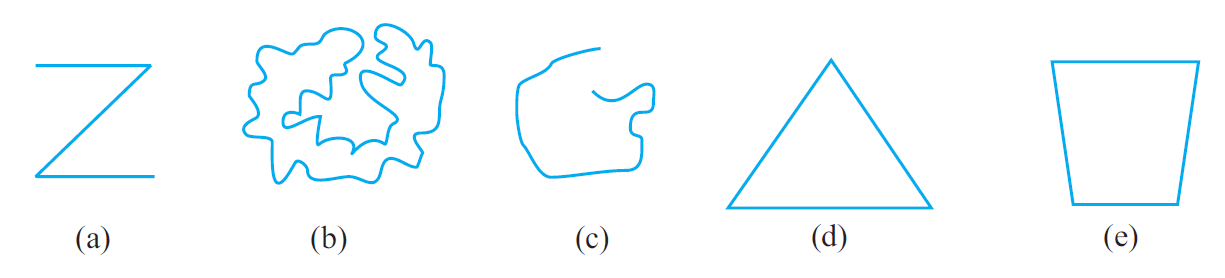a) Open curves
b) Closed curves
c) Open curves
d) Closed curves
e) Closed curves

Question 2: Draw rough diagrams to illustrate the following:
a) Open curve
b) Closed curve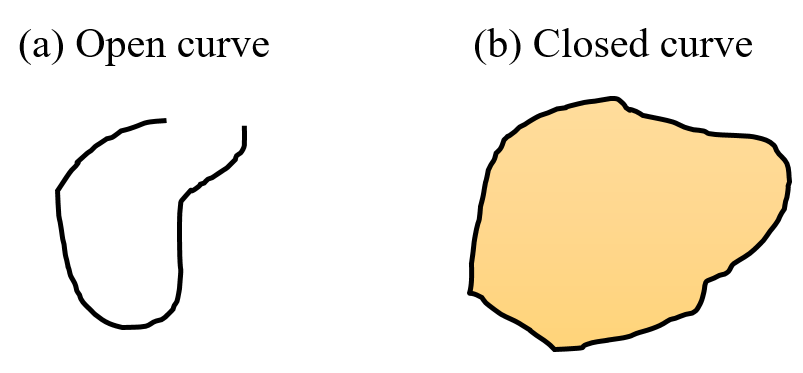Question 3: Draw any polygon and shade its interior.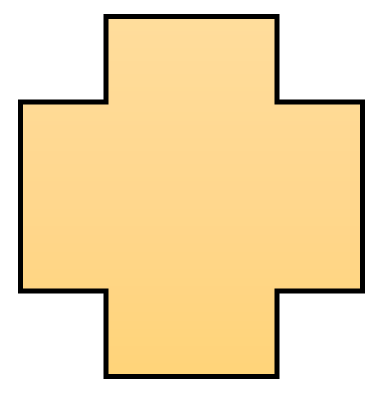Question 4: Consider the given figure and answer the questions: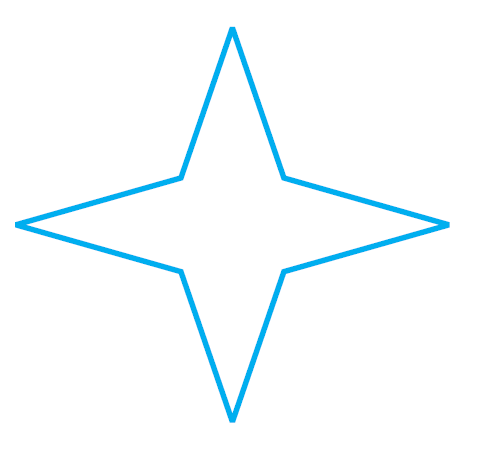a) Is it a curve?
b) Is it closed?

a) Yes, it is a curve.
b) Yes, it is closed.

Question 5: Illustrate, if possible, each one of the following with a rough diagram:
a) A closed curve that is not a polygon.
b) An open curve made up entirely of line segments.
c) A polygon with two sides.

a) Yes it is possible to illustrate a closed curve that is not a polygon.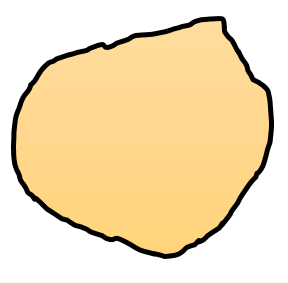b) Yes it is possible to illustrate a open curve made up entirely of line segments.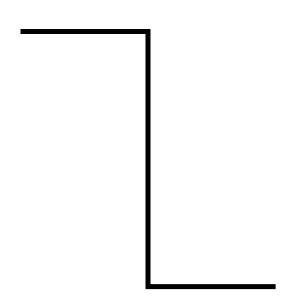c) No, it is not possible to illustrate a polygon with two sides. A polygon should have atleast three sides (triangle).